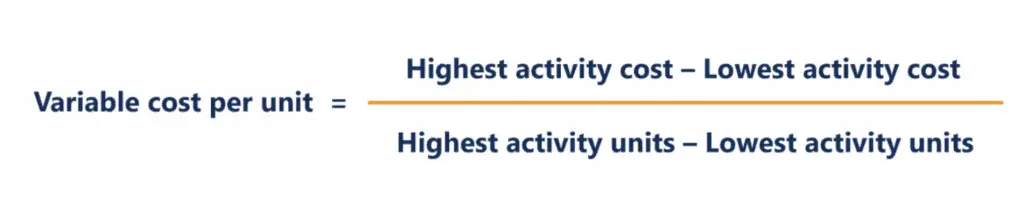# High Low Method

High low method is the mathematical method that cost accountant uses to separate between fixed and variable cost from mixed cost. We use the high low method when the cost cannot clearly separate due to its nature. Mixed cost is the combination of variable and fixed cost and it is also called “Semi Variable Cost”. It contains both fixed and variable costs.

High Low method will give us the estimation of fixed cost and variable cost, the result may be changed when the total unit and cost of both point change.

High low method uses the lowest production quantity and the highest production quantity and comparing the total cost at each production level. It uses only the lowest and highest production activities to estimate the variable and fixed cost, by assuming the production quantity and cost increase in linear. It ignores the other points of productions, so it may be an error when the cost does not increase in a linear graph. The two points are not representing the production cost at a normal level.

## High Low Method FormulaFixed Cost = Mixed Cost – Total variable cost

## High Low Method Example

Company A produces product X. During 20X9, the company has produced the following units and incur mixed cost:

 Month Units Mixed Cost January 1,000 13,000 February 1,200 14,000 March 1,500 15,500 April 900 12,500 May 1,000 13,000 June 1,300 14,500

Please calculate the fixed and variable cost per unit.

The highest units are in Marche 1,500 units with the highest cost \$ 15,500

The lowest units produce is in April 900 units with the lowest cost \$ 12,500

The variable cost per unit is:

Variable cost = (\$ 15,500 – \$ 12,500) / (1,500 units – 900 units)

= \$ 5 per units

Fixed cost = Mixed Cost – total variable cost

= \$ 15,500 – (1,500 units * \$ 5)

= \$ 8,000

Note: we can calculate the fixed cost from any level of activity from January to June as it will provide the same result.